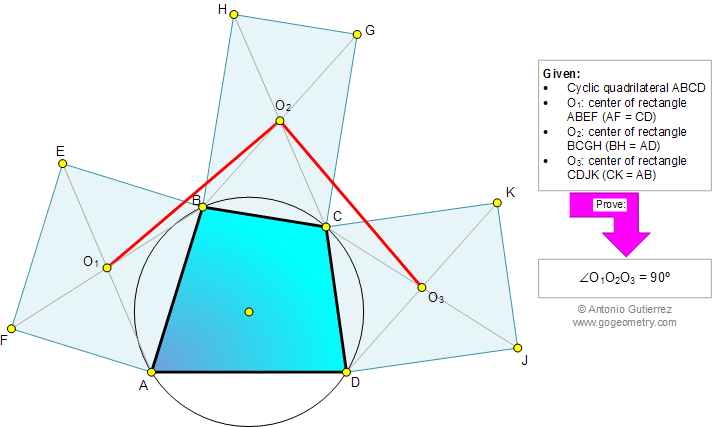###### Online Geometry Problem 866: Cyclic Quadrilateral, Circle, Rectangle, Center, Congruence, 90 Degrees. Level: High School, College, Mathematics Education
 The figure below shows a cyclic quadrilateral ABCD. ABEF, BCGH, and CDJK are rectangles of centers O1, O2, and O3, respectively, so that AF = CD, BH = AD, and CK = AB. Prove that the measure of angle O1O2O3 is 90 degrees.See also: Typography of problem 866.
 Home | Search | Geometry | Problems | All Problems | Open Problems | Visual Index | 10 Problems | 861-870 | Cyclic Quadrilateral | Circle | Rectangle | Congruence | Perpendicular lines, 90 degrees | Email | Solution / comment | By Antonio Gutierrez